## 9. Cotangents and tangents, cosecants and secants

### Cotangents

From the results obtained in the previous section we can easily deduce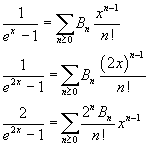Using the (3.9) to express the hyperbolic cotangent, we have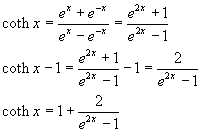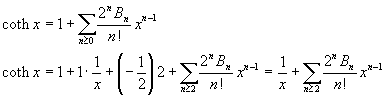If we explicitly write some addends of the sum we have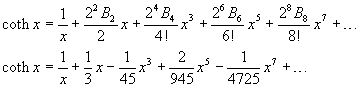Finally, using a more formal and compact notation: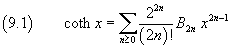The series expansion of the circular cotangent can be obtained from the (9.1), (7.21) and (7.22)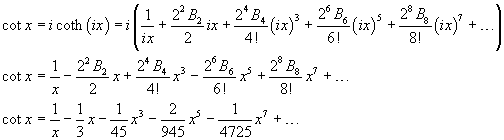In a more compact way: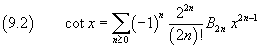### Tangents

To deduce the series expansion of the hyperbolic tangent we can use the equality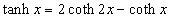In fact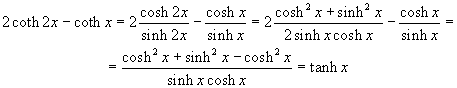So, using twice the series expansion of the hyperbolic cotangent, we get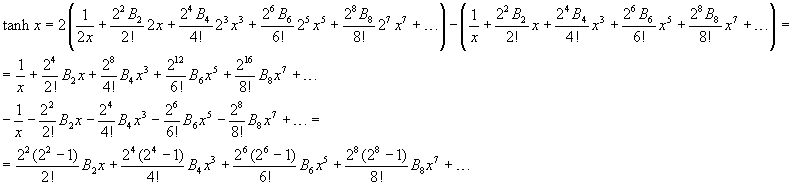If we calculate explicitly the coefficients, we haveIn a more compact way: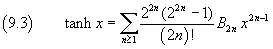To have the series expansion of the circular tangent we use the equality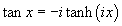We get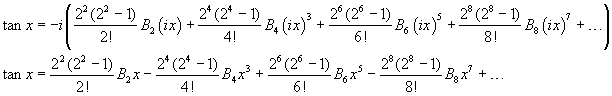If we calculate explicitly the coefficients, we have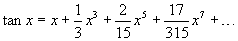In a more compact way: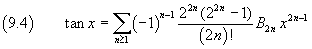### Cosecants

Since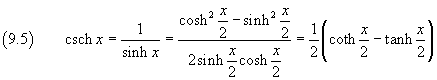the series expansion of the hyperbolic cosecant can be obtained using the series expansions of the hyperbolic tangent and cotangent. From (9.1) and (9.3) we get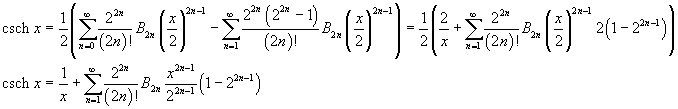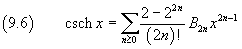More explicitly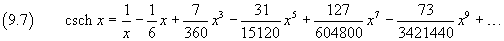From (7.22) e (9.7) we get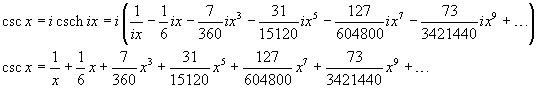and, in more compact way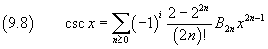### Secants

The hyperbolic secant can be expressed as a function of the exponentialThis expression of the hyperbolic secant coincides with that of the function s(x) defined in (8.2) of the previous page, then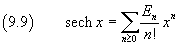where En is the n-th Euler's number. Since, for odd indices these numbers are zero, we can more economically write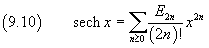From the Euler's formulas, the circular secant can be expressed as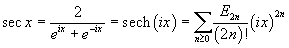The even powers of the imaginary unit oscillate between 1 and -1, then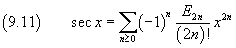Series expansions in WolframAlpha.